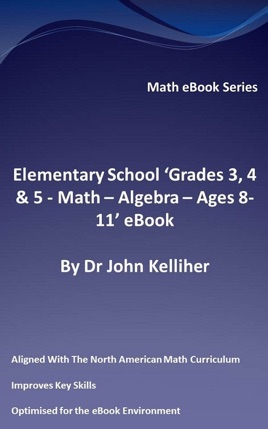• 25,00 kr

## Utgivarens beskrivning

This eBook introduces the subject of algebra, considers the inverse operators of addition and subtraction, the inverse operators of multiplication and division, introduce equations, consider the order of operations (precedence) and examines solving simple equations.This eBook is part of our range of Grades 3, 4 & 5 math eBooks that are aligned with the North American math curriculum.